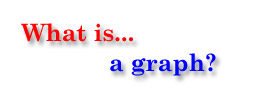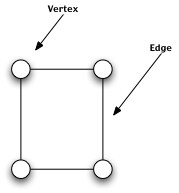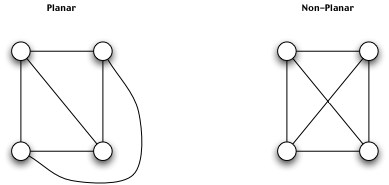A graph is simply a collection of vertices connected by edges. We can liken a graph to the design of a small, switched computer network in which the vertices are the computers and the edges are represented by the network cables connecting them. Another example may be seen in the airline system; airports are the vertices while direct flights between airports are the edges. One vertex of a graph is considered connected to another if they have an edge between them.For this project, I chose to look at a specific subclass of graphs known as planar graphs. A planar graph is a graph that can be drawn on a 2-dimentional plane such that no edges cross other edges.What is Graph Coloring?